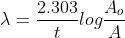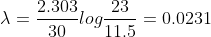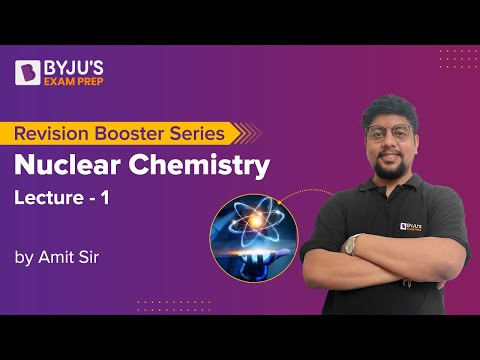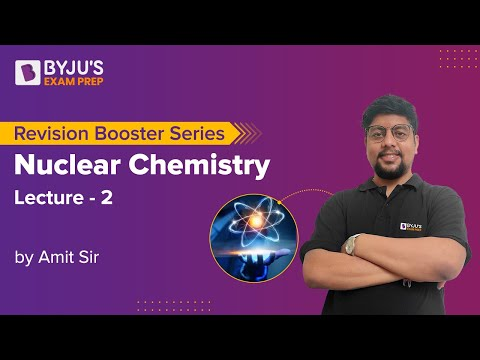Jet Set Go! All about Aeroplanes Jet Set Go! All about Aeroplanes

# Nuclear Chemistry Questions

Nuclear chemistry is the study of elements’ physical and chemical properties as they are affected by changes in the structure of the atomic nucleus. It also discusses nuclear energy and its applications. It is also known as radiochemistry, and it includes the study of the formation of elements in the universe, the development of radioactive drugs for diagnostic purposes, and many other applications.

 Definition: It is the study of the structure of atomic nuclei and the changes that occur within them.

## Nuclear Chemistry Questions with Solutions

Q-1:To sterilise surgical instruments, which of the following radioisotopes is used?

a) Iodine-131

b) Chromium-51

c) Iodine-123

d) Cobalt-60

Explanation: Cobalt-60 radioisotope is used to sterilise surgical instruments as well as to improve the safety and reliability of industrial fuel burners.

Q-2: Consider the following radioactive decay,

23892U→20682Pb

Find the total number of 𝞪-particles and 𝜷-particles.

Answer: Let us assume number of 𝞪-particles emitted are x and number of 𝜷-particles emitted are y.

23892U→20682Pb + x42He + y -10𝜷

Total mass number on the reactant side= Total mass number on product side

238= 4x+206

On solving,x= 8

Total atomic number on reactant side= Total atomic number on product side

92=82+2x-y

92= 82+2(8)-y

On solving, y= 6

Therefore, x+y= 14

Q-3: The type of radiation with the greatest ability to penetrate matter is

1. Alpha
2. Visible light
3. Gamma
4. 𝜷

Q-4: Which one of the following nuclides is most likely to decay by positron(𝜷+) emission is

a) 68Cu

b) 63Cu

c) 67Cu

d) 59Cu

Explanation: Those nucleus which have low value of n/p ratio undergoes positron(𝜷+) emission.

Nuclides

Neutrons,n= Mass number- protons

Protons

n/p ratio

68Cu

39

29

1.3448

63Cu

34

29

1.1724

67Cu

38

29

1.310

59Cu

30

29

1.03

Thus, the table clearly shows that nucleus 59Cu has the lowest n/p value and will therefore emit positron(𝜷+) .

Q-5: Write a balanced equation for the reaction of N14 with 𝞪-particles. Use shorthand notation to express the reaction.

Answer: The nucleus of nitrogen atom is converted into the nucleus of oxygen atom by 𝞪-particle(42He) and proton is also produced as a by-product. The reaction is shown below:

147N + 42He→ 178O + 11H

The above reaction can be expressed by short hand notation given below, in which the projectile and the liberating particle are expressed by their symbols in small brackets in between the parent and product nucleus.

Shorthand notation: 147N (𝞪,p)178O

Q-6: Differentiate between nuclear and chemical reactions.

Chemical Reactions

Nuclear Reactions

No new element is formed

New element is formed

Valence electrons of atoms participate in reaction.

Only the nucleus of atoms participates in reaction.

Balanced by the conservation of atoms

Balanced by the conservation of nuclear charge and mass number

Mass conservation is obeyed

Disobey mass conservation

Rate depends on external factors like temperature and the catalytic conditions

Rate is independent from any external condition

Q-7: Which of the following nuclear reactor components is used to slow down fast-moving neutrons without absorbing them?

a) Control rods

b) Coolant

c) Moderator

d) Fissile materials

Explanation: The functions of various parts of the nuclear reactor are:

a) Control rods: These are the rods of material which can absorb neutrons and hence control the fission reaction.

b) Coolant: These are the materials which transform the energy produced in the fission reaction into heat energy.

c) Moderator: It is used to slow down the fast moving neutrons without absorbing them.

d) Fissile materials are the nuclides which directly result in chain reaction on bombardment with slow neutrons.

Q-8: When a radioactive nucleus decays, it emits one alpha particle and two beta particles; the daughter nucleus is ________ of the parent nucleus.

a) Isobar

b) Isotope

c) Isoelectronic

d) Isotones

Explanation: Consider the case of a radioactive nucleus that decays and emits one alpha particle and two beta particles.

23892U→23492U + 42He + 2 -10𝜷

We can clearly see that the parent nuclei 23892U and daughter nuclei 23492U have the same atomic number(protons), therefore they both are isotopes.

Q-9: A radioactive sample has an initial activity of 23 dis/min. After 1/2h, the activity is 11.5 dis/min. How many atoms of the radioactive nuclide were present originally?[𝝺t1/2=0.69]Where, Ao is the initial activity and A is the activity at time,t

Given: Ao = 23 dis/min, A= 11.5 dis/min, t= ½= 30 minAlso, AO=𝝀No

Where, No is the number of atoms present initially.

23= 0.0231×No

No= 103

Q-10: The radioactive element Ra( Z=88) emits three alpha particles in succession. Deduce in which group the resulting element will be found?

Explanation: After emitting three alpha particles (42He) in succession, the radioactive element Ra(Z=88) yields an element with the atomic number 82(Pb). Pb is a member of Periodic Table Group 14.

Q-11: A radioactive nuclide’s half life is 20 years. If a sample of this nuclide has an activity of 6400 disintegrations per minute (dis/min) today, calculate its activity (dis/min) after 100 years.

A=Aoe-𝞴t

Where A is the activity at time, t

Ao is the activity initially

t= 100 years

𝝺 is the decay constant

𝝺 = 0.693/t1/2= 0.693/20= 0.03465 min-1

Substituting, Ao= 6400 dis/min , t= 100 min and 𝝺 = 0.03465 min-1 in A=Aoe-𝞴t, we get

A=6400e-0.03465×100

A= 200 dis/min

Q-12: What are magic numbers?

Answer: Nuclei with 2,8,20,50,82 or 126 protons or neutrons are exceptionally stable and have a larger number of stable isotopes than neighbouring nuclei in the periodic table. These numbers are called magic numbers. Nuclei with a magic number of protons as well as neutrons have notably high stabilities.

Q-13: Calculate the binding energy and binding energy per nucleon in kJ/mol of 1530P

Mass of 11H= 1.0078 amu

Mass of neutron= 1.0087 amu

Mass of 1530P = 29.978 amu

Answer: Binding energy = Δm(amu)× 931.5 Mev

Number of neutrons in 1530P= 15

Number of protons in 1530P= 15

Mass defect (Δm) = mass of neutron + mass of proton- mass of nucleus

Δm = (15×1.0087+ 15×1.0078-29.978)amu = 0.2695 amu

Binding energy = 0.2695 × 931.5 = 251.04 Mev

Binding energy per nucleon= B.E/number of nucleons = 251.04/29.978 = 8.37 Mev

Q-14: Mention the following radioisotopes’ applications: thorium-229, uranium-234, and iridium-192.

Thorium-229: Helps fluorescent lights last longer

Uranium-234: Used in dental fixtures like crowns and dentures to provide a natural colour and brightness

Iridium-192: Used to test the integrity of pipeline welds, boilers and aircraft parts.

Q-15: The nuclide 19879Au has a half life of 64.8 hours. How much of a 0.0100 g sample remains at the end of 1 day.

Answer: Half life, t1/2= 64.8 hours , t= 1day= 24 hours

We know that, t1/2= 0.693/𝞴

Decay constant,𝞴= 0.693/64.8 = 0.0107 hr-1

A= Aoe-𝞴t

= 0.01e-(0.0107×24)

=0.007735 g

## Practise Questions on Nuclear Chemistry

Q-1: In the following equation X is

24195Am + 24𝞪 → 97243Bk + X

a) 2 01n

b) 01n

c) 2 11H

d) 24He

Q-2:21584Po undergoes a 𝞪-emission, yielding element X, followed by 𝞫-emission, yielding element Y.

a) Write the valence shell electron configuration of Y.

b) Indicate the groups of the periodic table to which X and Y belong.

Q-3: In Boron neutron capture therapy, the initial boron isotope used and the particle generated after neutron capture respectively are:

a) 11B and 𝞪- particle

b) 10B and 𝞪- particle

c) 11B and 𝜷- particle

d) 10B and 𝜷- particle

Q-4: Radioactive decay is a process of the first order. Radioactive carbon decays with a half life of 5770 years in wood samples. What is the decay rate constant (in yr-1)? What fraction would remain after 11540 years?

Q-5: Complete the following nuclear reactions.

a) 73Li + 11H →

b) 23592U + 01n→ 13752A + 9740B + _______

c) 3482Se → 2 -10e + ____

d) 1327Al + ___→ 1328Al + 𝜸

Click the PDF to check the answers for Practice Questions.

## Nuclear Chemistry – Lecture 1## Nuclear Chemistry – Lecture 2